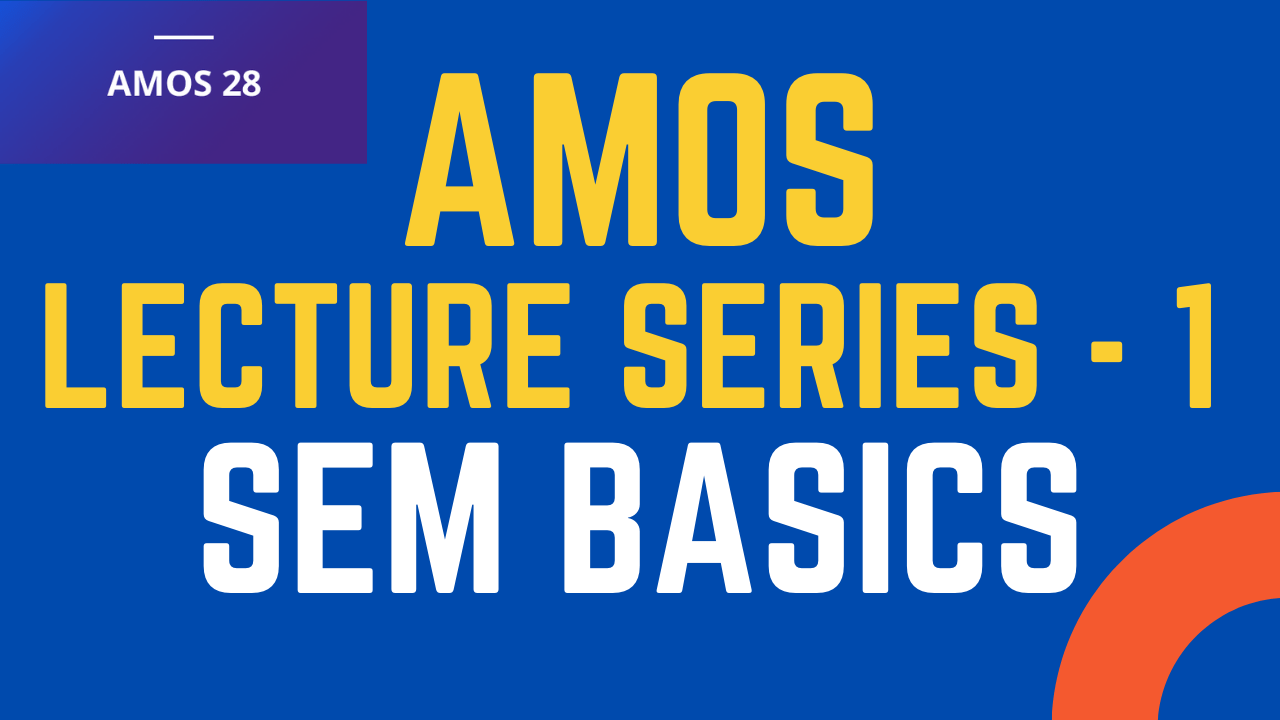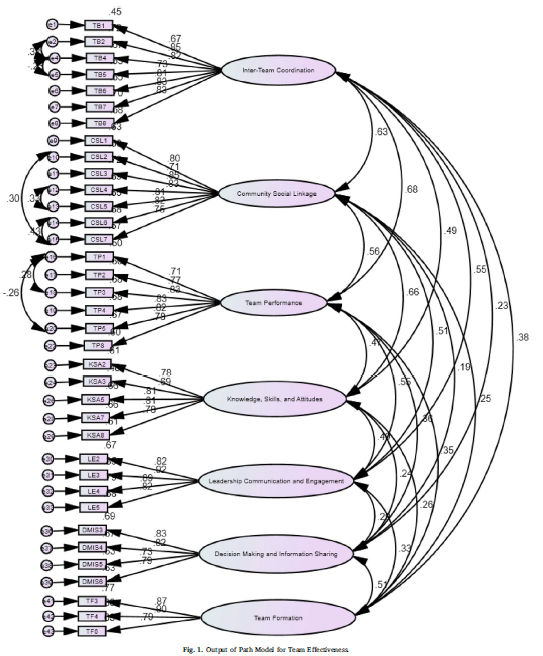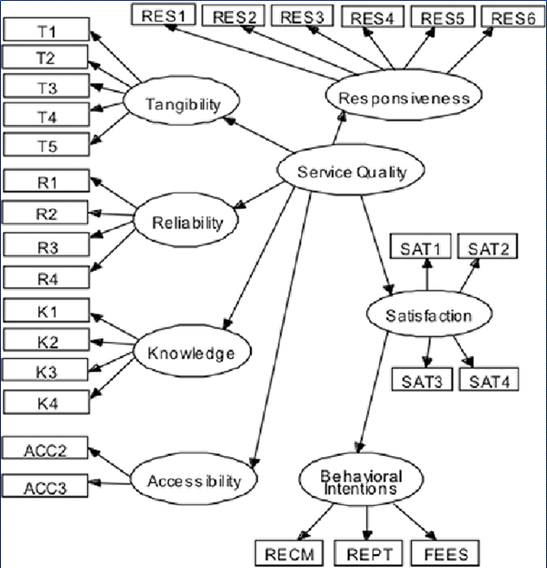# SPSS AMOS Lecture Series - The Basics

## SPSS AMOS - Lecture Series

The lecture series is focused on IBM SPSS AMOS. A second-generation structural equation modelling software that is an extension of IBM SPSS. The lecture series will focus in detail on the following

• Introduction to SEM
• Introduction to CFA
• Measurement and Structural Model
• Basic Measurement Model
• AMOS Output
• Improving Model Fit
• Assessing Reliability and Validity
• Basic Structural Model
• Reporting Measurement and Structural Model
• Mediation Analysis
• Reporting Mediation Analysis
• Moderation Analysis
• Reporting Moderation Analysis### Learn SPSS AMOS

The tutorial focuses on the basics of Structural Equation Modelling and a breif overview of modelling in AMOS

## What is Structural Equation Modelling?

Structural equation modelling (SEM) is a set of statistical techniques used to measure and analyze the relationships of observed and latent variables.

A Structural Equation Model comprises of Measurement and Structural Model

## Measurement Model - Confirmatory Factor Analysis

Confirmatory factor analysis (CFA) is a statistical technique that analyzes how well your indicators measure your unobserved constructs and if your unobserved constructs are uniquely different from one another.

The first step in SEM using AMOS is to draw a Measurement Model for Confirmatory Factor Analysis.

All the constructs in the study are drawn and then covaried with each other to perform CFA.

Then, the model is assessed for Model Fit, and the Reliability and Validity of the Constructs is Assessed.## Structural Model

Once, the measurement model assessment is complete, the next step is structural model assessment that refers to testing the significance of hypothesized paths.## Key Terminologies

• Constructs: measure theoretical concepts that are abstract, complex, and cannot be directly observed by means of (multiple) items. Constructs are represented in path models as circles or ovals and are also referred to as latent variables.
• Indicators: these are directly measured observations (raw data), also referred to as either items or manifest variables, which are represented in path models as rectangles. They are also available data (e.g., responses to survey questions or collected from company databases) used in measurement models to measure the latent variables.
• Factor loadings: the bivariate correlations between a construct and the indicators. They determine an item’s absolute contribution to its assigned construct. Loadings are of primary interest in the evaluation of reflective measurement models but are also interpreted when formative measures are involved.
• Latent variables: elements of a structural model that are used to represent theoretical concepts in statistical models. A latent variable that only explains other latent variables (only outgoing relationships in the structural model) is called exogenous, while latent variables with at least one incoming relationship in the structural model are called endogenous. Also see Constructs.
• Critical t value: the cutoff or criterion on which the significance of a coefficient is determined. If the empirical t value is larger than the critical t value, the null hypothesis of no effect is rejected.